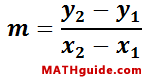Classifying Parallelograms Using Slope
View the Lesson | MATHguide homepage Updated January 25th, 2022

Given:Quadrilateral A(-4, 9), B(10, 3), C(13, 10), D(-1, 16)Problem:Determine the slope of all sides and the two diagonals to best classify quadrilateral ABCD.
Sides
 mAB =mBC =mCD =mAD =Diagonals
 mAC =mBD =Classification: